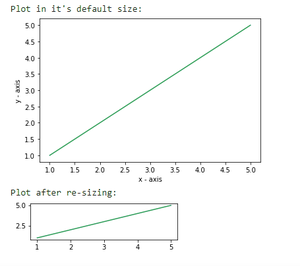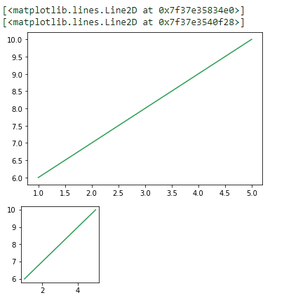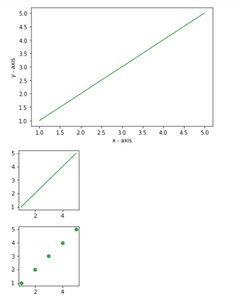Related Articles

# Change plot size in Matplotlib – Python

• Difficulty Level : Basic
• Last Updated : 26 Nov, 2020

Prerequisite: Matplotlib

Plots are an effective way of visually representing data and summarizing it in a beautiful manner. However, if not plotted efficiently it seems appears complicated. In python’s matplotlib provides several libraries for the purpose of data representation.

While making a plot it is important for us to optimize its size. Here are various ways to change the default plot size as per our required dimensions or resize a given plot.

Method 1: Using set_figheight() and set_figwidth()

For changing height and width of a plot set_figheight and set_figwidth are used

## Python3

 `# importing the matplotlib library``import` `matplotlib.pyplot as plt`` ` `# values on x-axis``x ``=` `[``1``, ``2``, ``3``, ``4``, ``5``]``# values on y-axis``y ``=` `[``1``, ``2``, ``3``, ``4``, ``5``]`` ` `# naming the x and y axis``plt.xlabel(``'x - axis'``)``plt.ylabel(``'y - axis'``)`` ` `# plotting a line plot with it's default size``print``(``"Plot in it's default size: "``)``plt.plot(x, y)``plt.show()`` ` `# plotting a line plot after changing it's width and height``f ``=` `plt.figure()``f.set_figwidth(``4``)``f.set_figheight(``1``)`` ` `print``(``"Plot after re-sizing: "``)``plt.plot(x, y)``plt.show()`

Output:Method 2: Using figsize

figsize() takes two parameters- width and height (in inches). By default the values for width and height are 6.4 and 4.8 respectively.

Syntax:

plt.figure(figsize=(x,y))

Where, x and y are width and height respectively in inches.

## Python3

 `import` `matplotlib.pyplot as plt`` ` `# values on x and y axis``x ``=` `[``1``, ``2``, ``3``, ``4``, ``5``]``y ``=` `[``6``, ``7``, ``8``, ``9``, ``10``]`` ` `# plot in it's default size``display(plt.plot(x, y))`` ` `# changing the size of figure to 2X2``plt.figure(figsize``=``(``2``, ``2``))``display(plt.plot(x, y))`

Output:output screenshot

Method 3: Changing the default rcParams

We can permanently change the default size of a figure as per our needs by setting the figure.figsize.

## Python3

 `# importing the matplotlib library``import` `matplotlib.pyplot as plt`` ` `# values on x-axis``x ``=` `[``1``, ``2``, ``3``, ``4``, ``5``]``# values on y-axis``y ``=` `[``1``, ``2``, ``3``, ``4``, ``5``]`` ` `# naming the x axis``plt.xlabel(``'x - axis'``)``# naming the y axis``plt.ylabel(``'y - axis'``)`` ` `# plotting a line plot with it's default size``plt.plot(x, y)``plt.show()`` ` `# changing the rc parameters and plotting a line plot``plt.rcParams[``'figure.figsize'``] ``=` `[``2``, ``2``]`` ` `plt.plot(x, y)``plt.show()`` ` `plt.scatter(x, y)``plt.show()`

Output:Attention geek! Strengthen your foundations with the Python Programming Foundation Course and learn the basics.

To begin with, your interview preparations Enhance your Data Structures concepts with the Python DS Course. And to begin with your Machine Learning Journey, join the Machine Learning – Basic Level Course

My Personal Notes arrow_drop_up